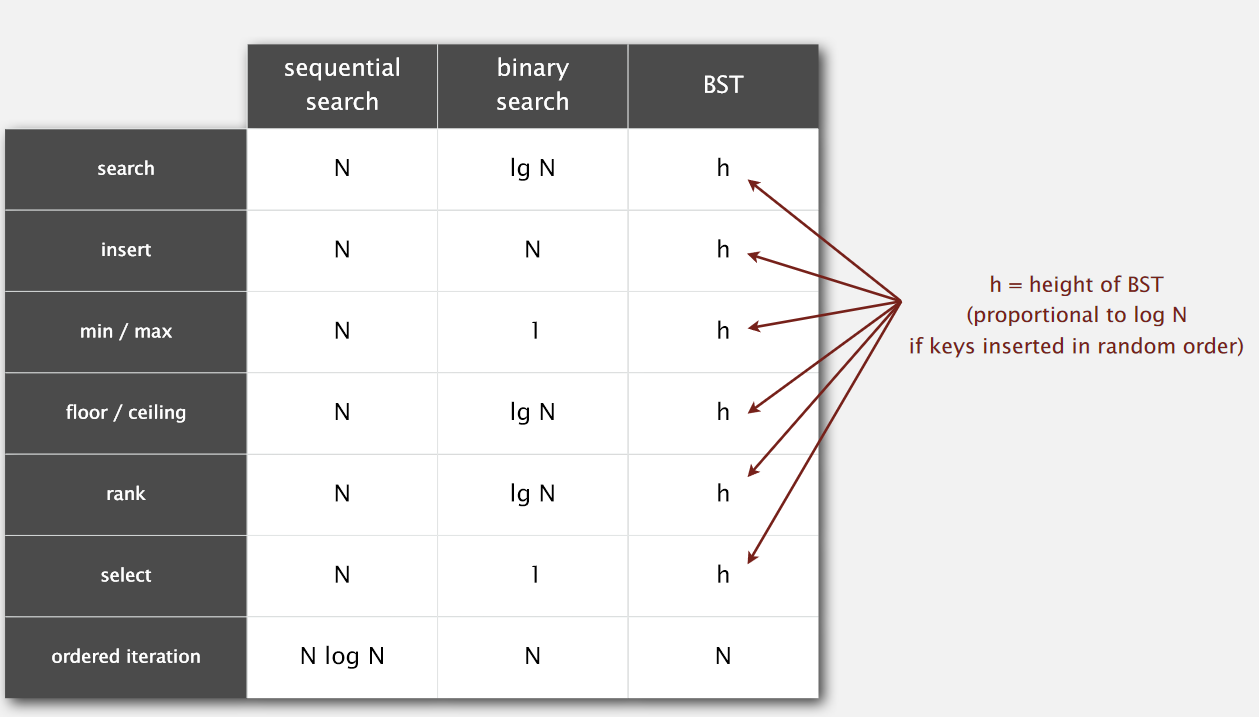# 符号表ST（3）——二叉查找树 （附动画）

2016-05-14   |     |     |

# 1 二叉查找树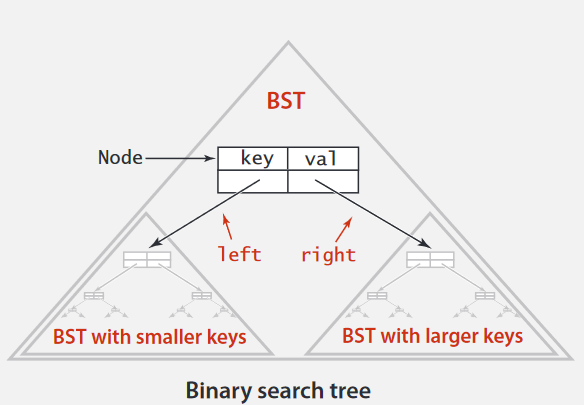## 1.1 代码框架

``````public class BST<Key extends Comparable<Key>, Value>
{
private Node root;
private class Node{}
public void put(Key key, Value val){}
public Value get(Key key){}
public void delete(Key key){}
public Iterable<Key> iterator(){}
}
``````

## 1.2 节点表示

``````private class Node {
private Key key;
private Value val;
private Node left, right;
public Node(Key key, Value val) {
this.key = key;
this.val = val;
}
}
``````

## 1.3 取得操作

• 如果 小于 ，左孩子
• 如果 大于，右孩子

• 如果找到，返回
• 如果为null，则取得失败

``````
public Value get(Key key)
{
Node x = root;
while(x != null)
{
int cmp = key.compareTo(x.key);
if(cmp < 0)
x = x.left;
else if(cmp > 0)
x = x.right;
else if(cmp == 0)
return x.val;
}
return null;
}
``````

## 1.4 插入操作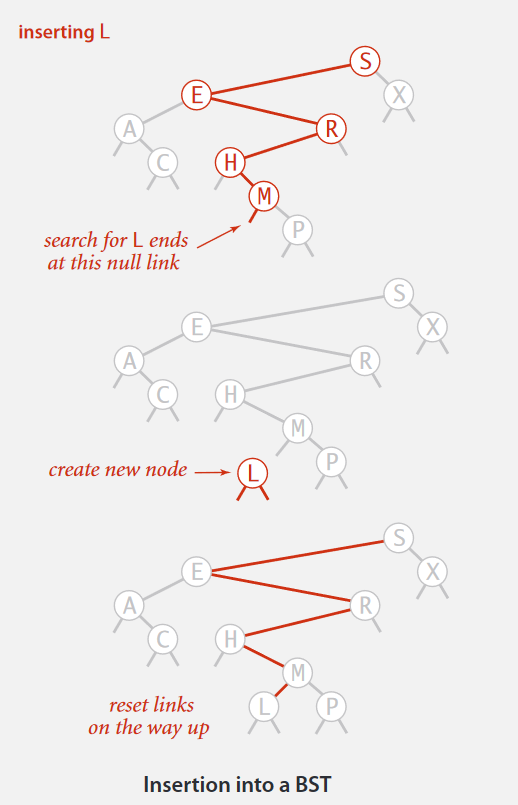`root = put(root, key, val)`

; 把root也结合进去了。

``````public void put(Key key, Value val) {
root = put(root, key, val);
}

private Node put(Node x, Key key, Value val) {
if (x == null) {
return new Node(key, val);
}
int cmp = key.compareTo(x.key);
if (cmp < 0) {
x.left = put(x.left, key, val);
} else if (cmp > 0) {
x.right = put(x.right, key, val);
} else if (cmp == 0) {
x.val = val;
}
return x;
}
``````

``````public void put(Key key,Value val)
{
Node x = root;
if(x == null)
x = new Node(key,val);
while(true)
{
int cmp = key.compareTo(x.key);
if(cmp < 0)
{
if(x.left != null)
x = x.left;
x.left = new Node(key,val);
return ;
}
if(cmp > 0)
{
if(x.right != null)
x = x.right;
x.right = new Node(key,val);
return ;
}
if(cmp == 0){
x.val = val;
return ;
}
}
}
``````

## 1.5 动画演示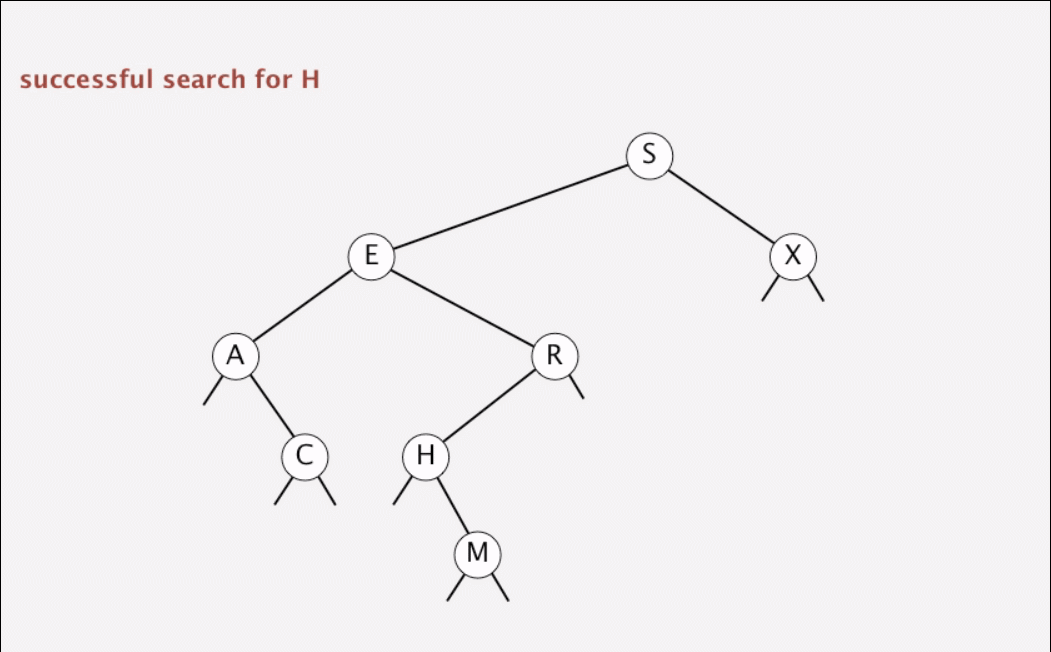## 1.6 二叉树的形状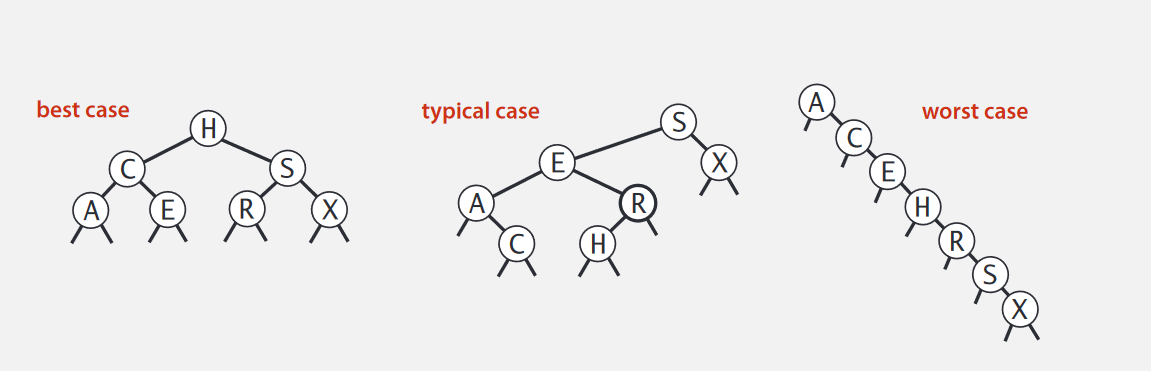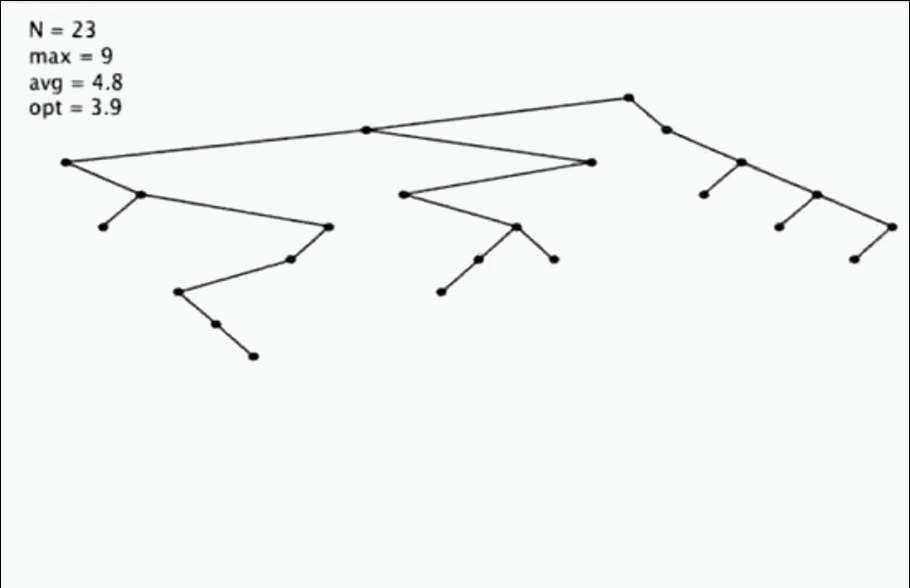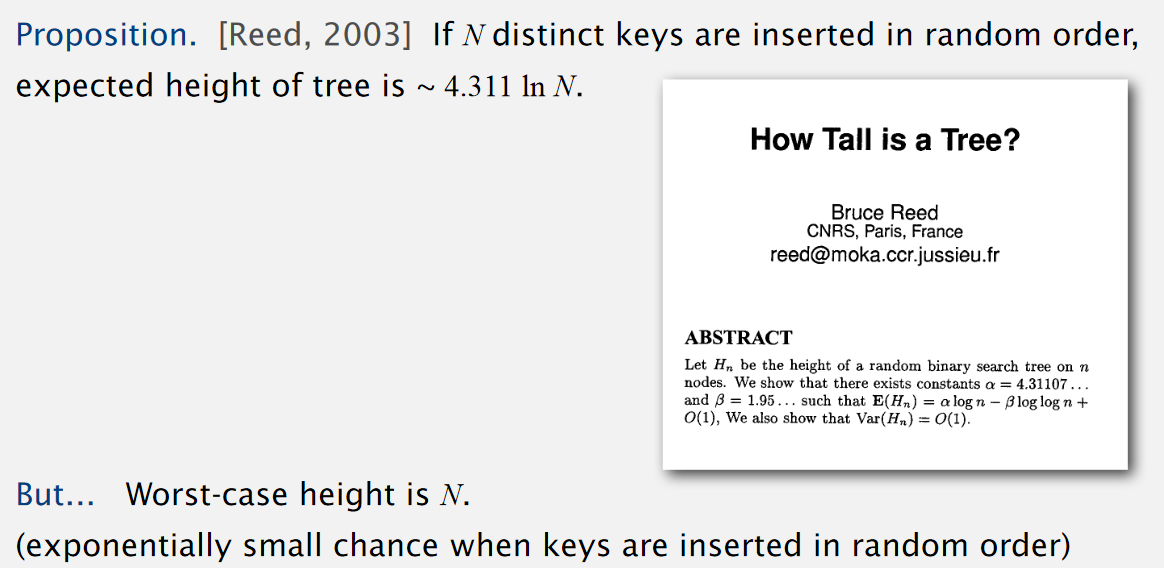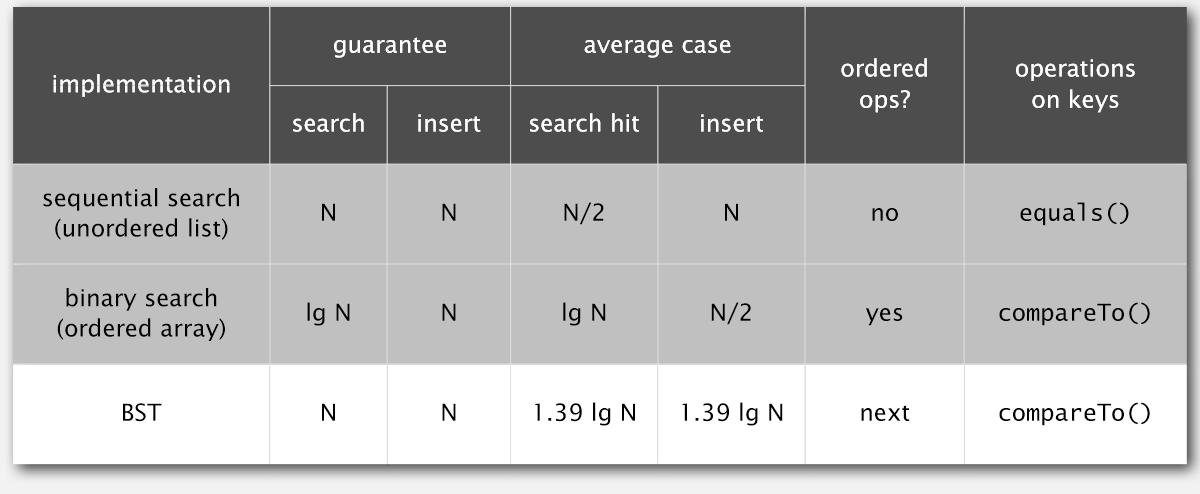# 2. 有序操作

## 2.1 最大最小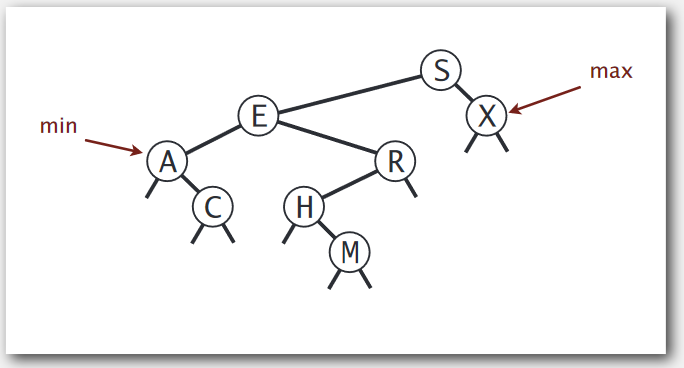## 2.2 floor和ceiling

floor：找到比key小的最大key ceiling：找到比key大的最小key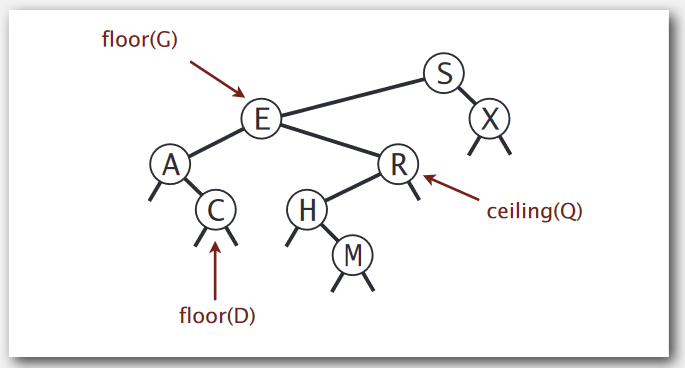## 2.2.1 floor操作：（递归操作）

• 条件一：首先前提条件是比给定的Key`小`
• 条件二：然后再在这些`小`值中找`最大`的一个。

• 如果k == `root.key` `floor` = `root.key`
• 如果k < `root.key``左子树`继续查找（杂没比我小的）

• 如果k > `root.key` 记录`root`（终于找到一个比我小的了，赶紧记下来）

• 如果`右边子树`遇到一个`小于`k的，就继续（看还有么有更大的`小`值）
• 否则，就是`root` （看来没有比你大的了）
```java

public Key floor(Key key)
{

``````  Node x = floor(root,key);
if(x == null) return null;
return x.key;
``````

}
private Node floor(Node x,Key key)
{

``````  if(x == null)   return x;
int cmp = key.compareTo(x.key);
if (cmp < 0)   return floor(x.left, key);
if (cmp == 0)   return x;
Node f = floor(x.right, key);
if (f == null)
return x;
else
return f;
``````

}
```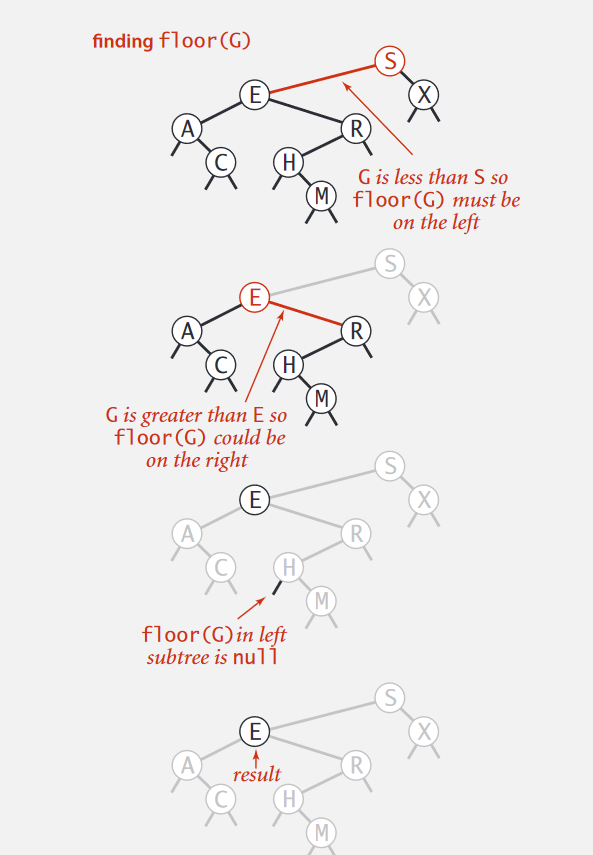## 2.2.2 ceiling操作：（递归操作）

• 如果k == `root.key` `floor` = `root.key`
• 如果k > `root.key``右子树`继续查找（杂没比我大的?）

• 如果k < `root.key` 记录`root`（终于找到一个比我大的了，赶紧记下来）

• 如果`左边子树`遇到一个`大于`k的，就继续（看还有么有更小的`大`值）
• 否则，就是`root`（看来没有比你小的了）
```java

public Key ceil(Key key) {

``````  Node x = ceil(root, key);
if (x == null) {
return null;
}
return x.key;
``````

}

private Node ceil(Node x, Key key) {

``````  if (x == null) {
return x;
}
int cmp = key.compareTo(x.key);
if (cmp > 0) {
return ceil(x.right, key);
}
if (cmp == 0) {
return x;
}
Node f = ceil(x.left, key);
if (f == null) {
return x;
} else {
return f;
}
``````

}
```

## 2.3 子树统计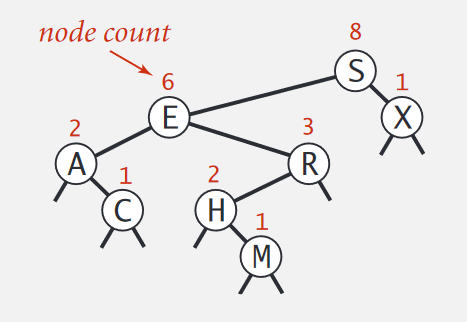``````private class Node
{
private Key key;
private Value val;
private Node left;
private Node right;
private int count;  //加这里
}

public int size()
{
return size(root);
}
private int size(Node x)
{
if (x == null) return 0;
return x.count;  //加这里
}

private Node put(Node x, Key key, Value val)
{
if (x == null) return new Node(key, val, 1);
int cmp = key.compareTo(x.key);
if (cmp  < 0) x.left  = put(x.left,  key, val);
else if (cmp  > 0) x.right = put(x.right, key, val);
else if (cmp == 0) x.val = val;
x.count = 1 + size(x.left) + size(x.right);//加这里
return x;
}
``````

## 2.4 rank()

rank的功能就是返回键小于key的个数。有三种情况：

• root == key 左孩子`count`（因为只有左孩子小于它）
• root < key 左孩子`count`+1（自己）+ 右孩子继续找
• root > key 左孩子继续找（给定key还没找到比自己小的呢）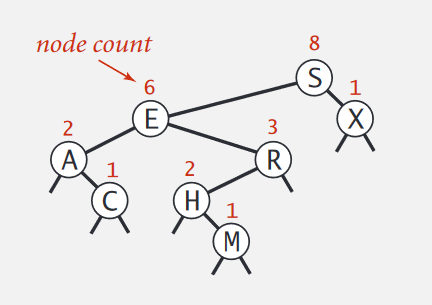``````public int rank(Key key)
{
return rank(root,key);
}
private int rank(Node x,Key key)
{
if(x == null) return 0;
int cmp = key.compareTo(x.key);
if(cmp > 0)
return 1 + size(x.left) + rank(x.right,key);
else if(cmp == 0)
return size(x.right);
return rank(x.left,key);
}
``````

## 2.5 有序遍历

• 遍历左孩子
• 把自己key插入队列
• 遍历右孩子

``````
public Iterable<Key> keys()
​    {
​        Queue<Key> q = new Queue<Key>();
​        inorder(root, q);
​        return q;
​    }
​    private void inorder(Node x,Queue<Key> q)
​    {
​        if(x == null)
​            return ;
​        inorder(x.left, q);
​        q.enqueue(x.key);
​        inorder(x.right,q);
​    }
``````

# 3 总结